Ex 3.2

Chapter 3 Class 11 Trigonometric Functions
Serial order wise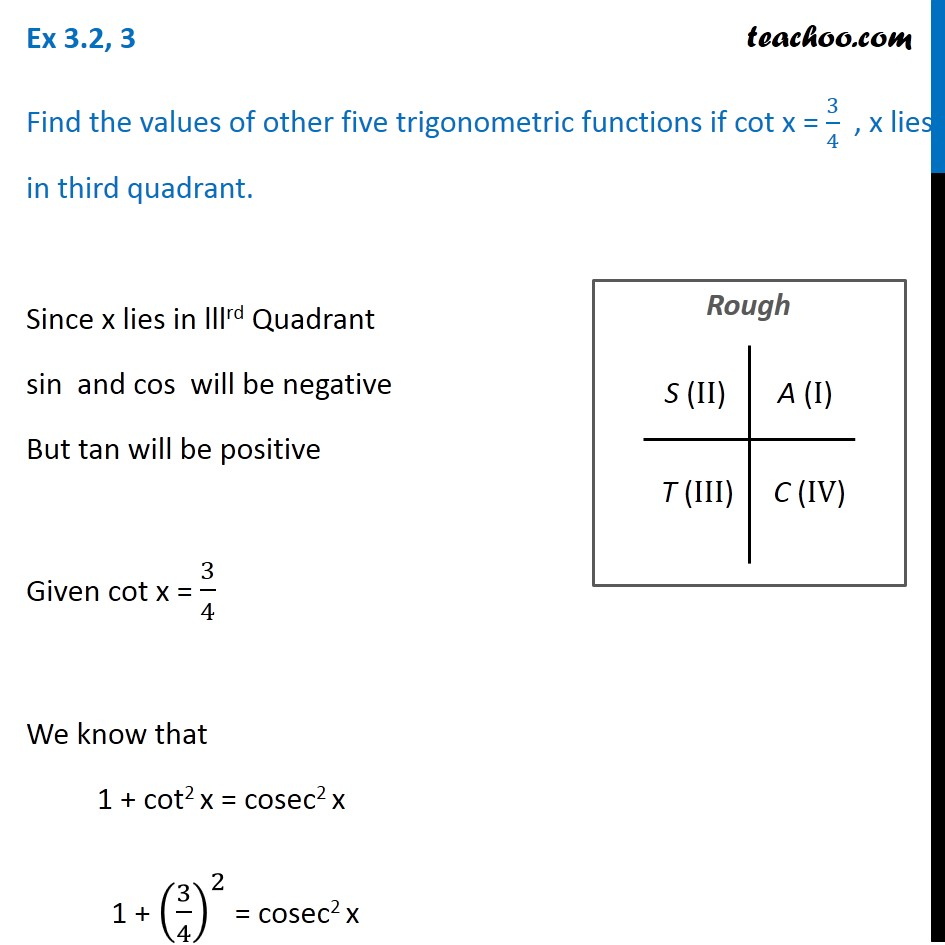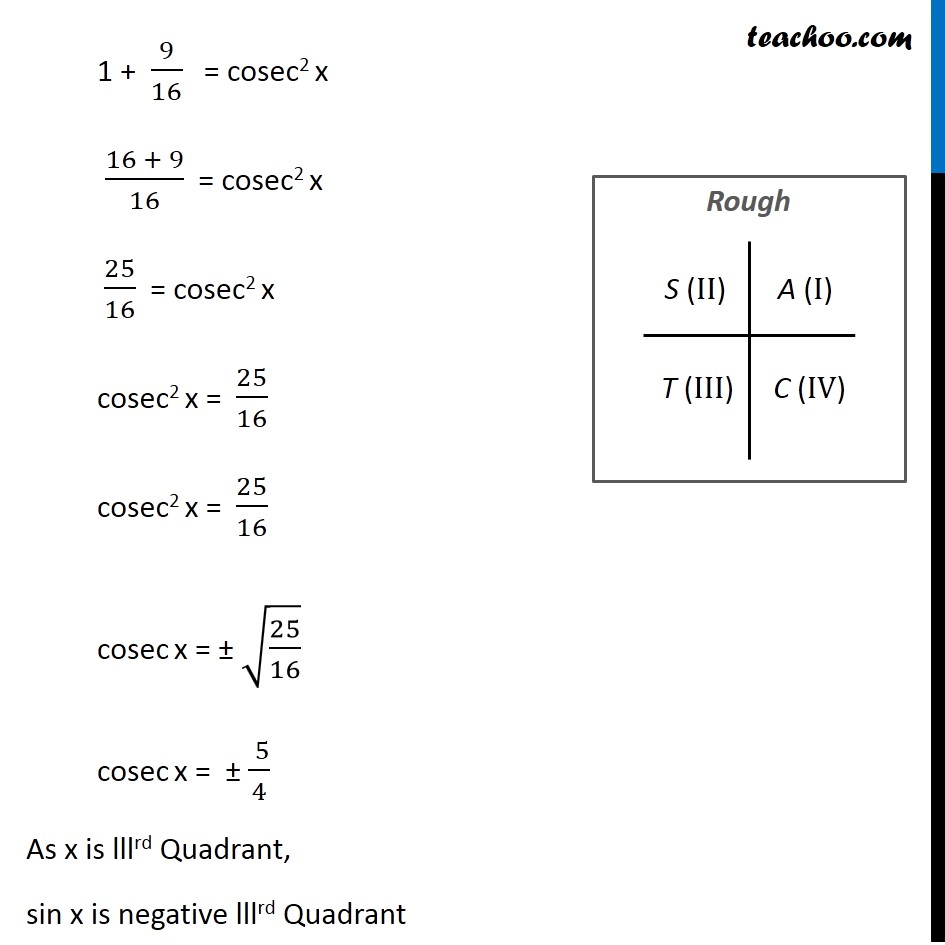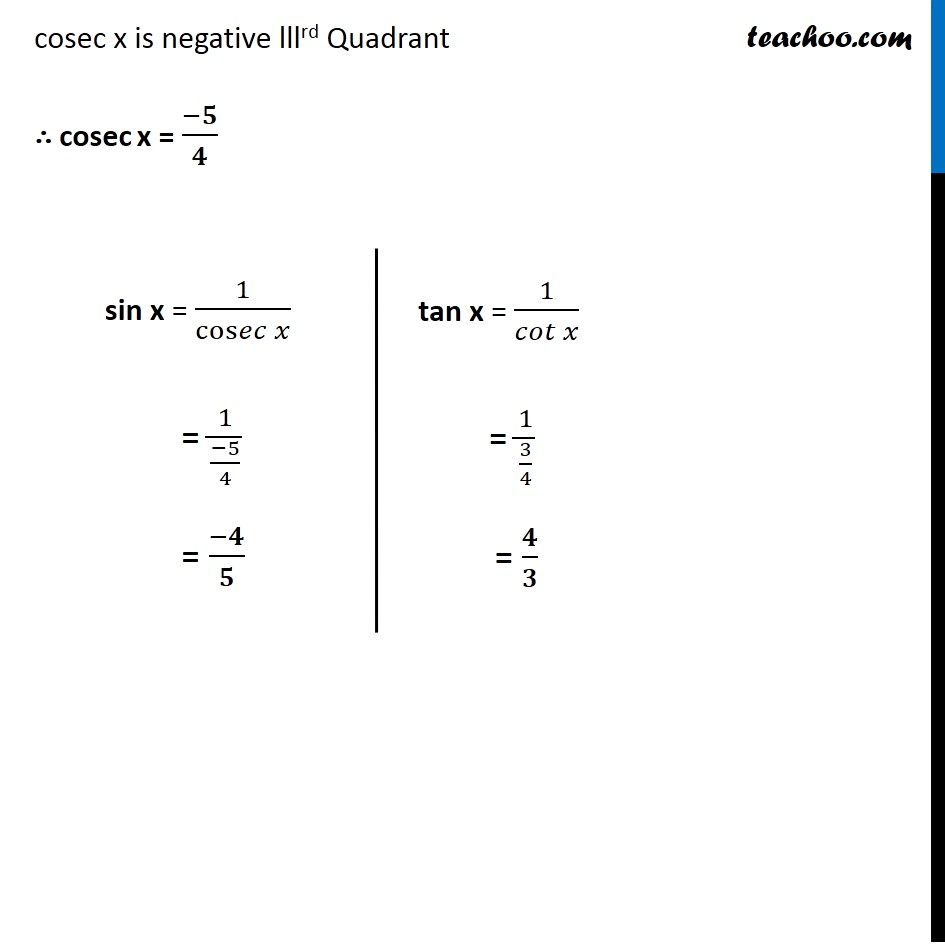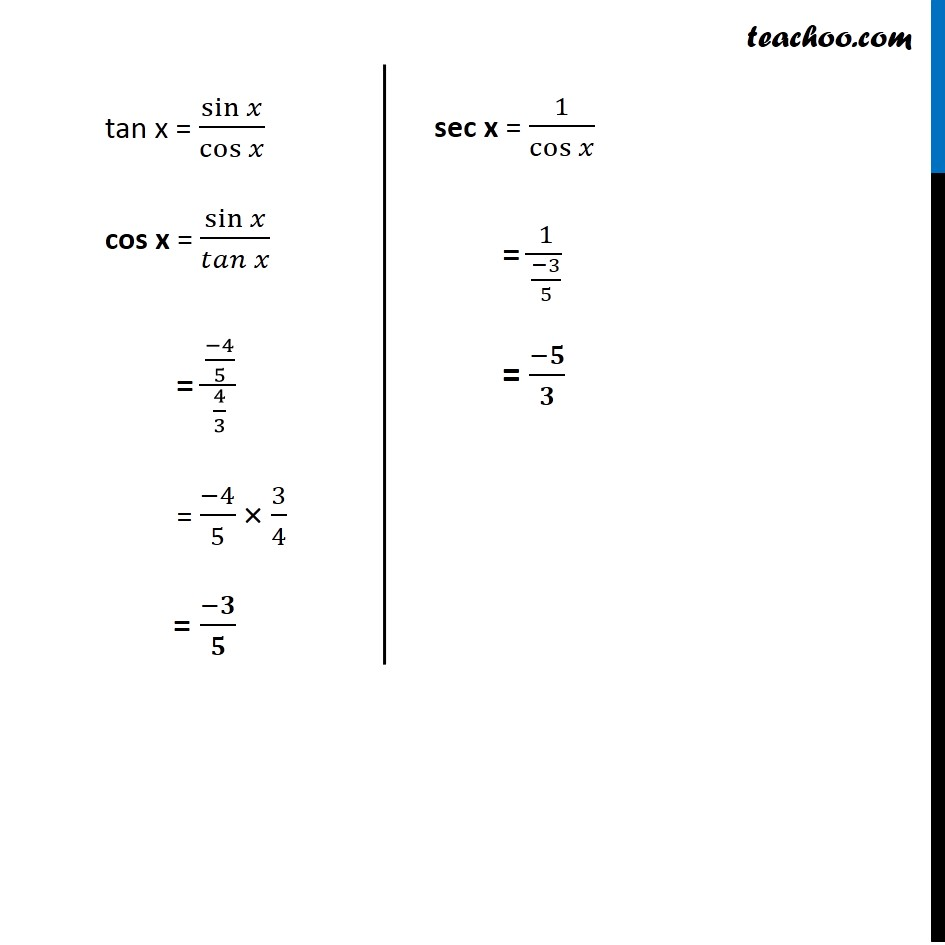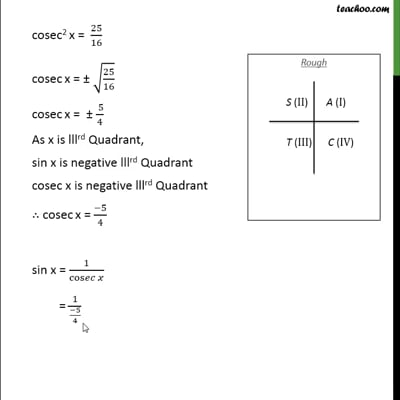This video is only available for Teachoo black users

Maths Crash Course - Live lectures + all videos + Real time Doubt solving!

### Transcript

Ex 3.2, 3 Find the values of other five trigonometric functions if cot x = 3/4 , x lies in third quadrant. Since x lies in lllrd Quadrant sin and cos will be negative But tan will be positive Given cot x = 3/4 We know that 1 + cot2 x = cosec2 x 1 + (3/4)^2 = cosec2 x 1 + 9/16 = cosec2 x (16 + 9)/16 = cosec2 x 25/16 = cosec2 x cosec2 x = 25/16 cosec2 x = 25/16 cosec x = ± √(25/16) cosec x = ± ( 5)/4 As x is lllrd Quadrant, sin x is negative lllrd Quadrant cosec x is negative lllrd Quadrant ∴ cosec x = (−𝟓)/𝟒 sin x = 1/cos𝑒𝑐⁡𝑥 = 1/((−5)/4) = (−𝟒)/𝟓 tan x = 1/(𝑐𝑜𝑡 𝑥) = 1/(3/4) = 𝟒/𝟑 tan x = sin⁡𝑥/cos⁡𝑥 cos x = sin⁡𝑥/𝑡𝑎𝑛⁡𝑥 = ((−4)/5)/(4/3) = (−4)/5 × 3/4 = (−𝟑)/𝟓 sec x = 1/cos⁡𝑥 = 1/((−3)/5) = (−𝟓)/𝟑